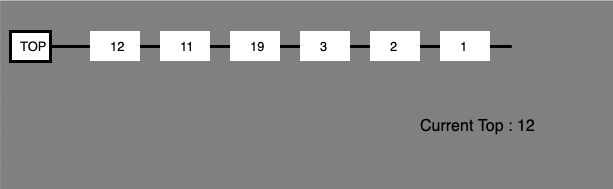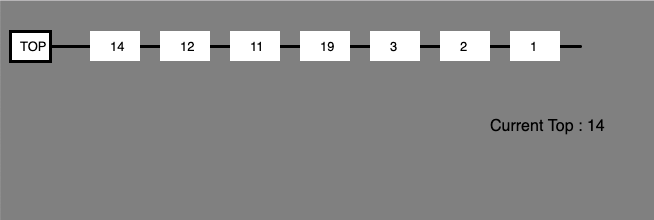p5.js | Push Operation on Stack

• Last Updated : 04 Oct, 2021

What is Stack?
The stack is a linear data structure like an array in which only the last inserted element can be accessed. This data structure works over the principle Last In First Out (LIFO). It is very fast since it has to access only last added element so it requires constant time to do so having complexity O(1)
Stacks should be used over arrays when we need to work with data in the LIFO form. Push operation on the stack is used to adds an item in the stack. If the stack is full, then it is said to be an Overflow condition.Basic skeleton of stack: Below example run using “\$node skeleton.js” command to get basic stack skeleton.

javascript

 // Define Stack functionfunction Stack(array) {    this.array = [];    if (array) this.array = array;} // Add Get Buffer property to object// constructor which slices the arrayStack.prototype.getBuffer = function() {    return this.array.slice();} // Add isEmpty properties to object constructor// which returns the length of the arrayStack.prototype.isEmpty = function() {    return this.array.length == 0;} // Instance of the stack classvar stack1 = new Stack(); // Stack { array: [] } console.log(stack1);

Example: This example describe the push operation on stack.

javascript

          Stack push operation                               <script>         // Define Stack function        function Stack(array) {            this.array = [];            if (array) this.array = array;        }                 // Add Get Buffer property to object        // constructor which slices the array        Stack.prototype.getBuffer = function() {            return this.array.slice();        }                 // Add isEmpty properties to object constructor        // which returns the length of the array        Stack.prototype.isEmpty = function() {            return this.array.length == 0;        }                 // Instance of the stack class        var stack1 = new Stack(); //Stack { array: [] }                 // Add Push property to object constructor which        // push elements to the array        Stack.prototype.push = function(value) {            this.array.push(value);        }                 function setup() {                     // Create Canvas of size display width * 300            createCanvas(displayWidth, 300);        }                 function draw() {                     // Set background color            background("grey");                         // Set stroke weight            strokeWeight(3);                         // Set stroke color            stroke('black');            line(10, 45, 90, 45);            rect(10, 30, 40, 30);            noStroke();            text("TOP", 20, 50);                     // Display stack            for(var i = stack1['array'].length-1; i >= 0; i--) {                var p = 10;                translate(70, 0);                strokeWeight(3);                stroke('black');                line(10+p, 45, p+80, 45);                noStroke();                rect(10+p, 30, 40+p, 30);                text(stack1['array'][i], 40, 50);                p +=10;            }        }                 // Call to push operation        stack1.push(1);        stack1.push(2);        stack1.push(3);        stack1.push(19);        stack1.push(11);        stack1.push(12);    </script></body> </html>

Output:After Pushing ’14’ by calling stack1.push(14) function to the top of stack.Hey geek! The constant emerging technologies in the world of web development always keeps the excitement for this subject through the roof. But before you tackle the big projects, we suggest you start by learning the basics. Kickstart your web development journey by learning JS concepts with our JavaScript Course. Now at it's lowest price ever!

My Personal Notes arrow_drop_up